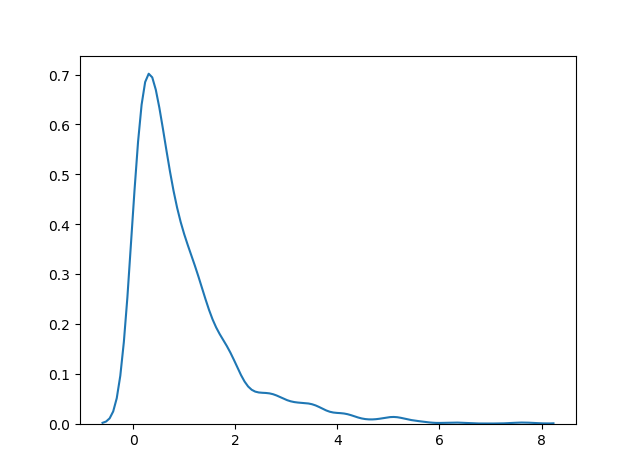# Exponential Distribution

## Exponential Distribution

Exponential distribution is used for describing time till next event e.g. failure/success etc.

It has two parameters:

`scale` - inverse of rate ( see lam in poisson distribution ) defaults to 1.0.

`size` - The shape of the returned array.

### Example

Draw out a sample for exponential distribution with 2.0 scale with 2x3 size:

from numpy import random

x = random.exponential(scale=2, size=(2, 3))

print(x)
Try it Yourself »

## Visualization of Exponential Distribution

### Example

from numpy import random
import matplotlib.pyplot as plt
import seaborn as sns

sns.distplot(random.exponential(size=1000), hist=False)

plt.show()

### ResultTry it Yourself »

## Relation Between Poisson and Exponential Distribution

Poisson distribution deals with number of occurences of an event in a time period whereas exponential distribution deals with the time between these events.

W3Schools is optimized for learning and training. Examples might be simplified to improve reading and learning. Tutorials, references, and examples are constantly reviewed to avoid errors, but we cannot warrant full correctness of all content. While using W3Schools, you agree to have read and accepted our terms of use, cookie and privacy policy.